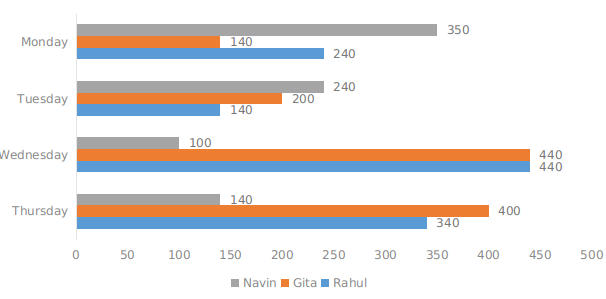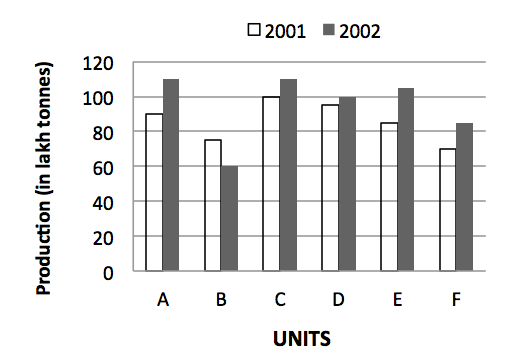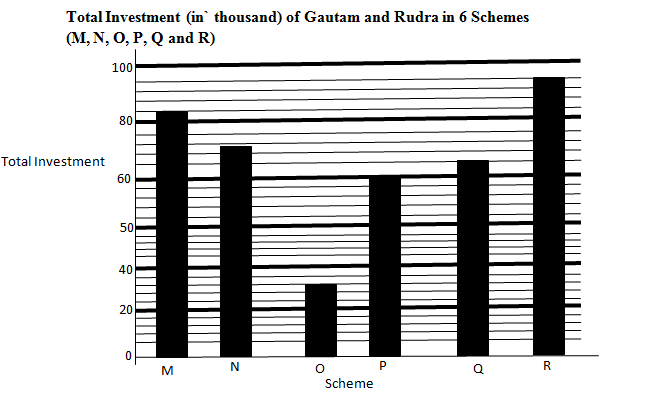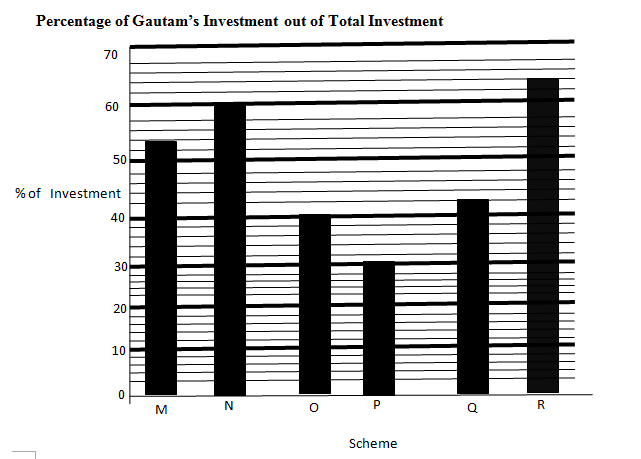0
64

Here you can download CMAT 2022 – important Bar Graph Questions PDF by Cracku. Very Important Bar Graph Questions for CMAT 2022 based on asked questions in previous exam papers. These questions will help your CMAT preparation. So kindly download the PDF for reference and do more practice.

Take Free CMAT mock tests here

Instructions

Instructions: Study the following bar-graph carefully and answer the following questions. Earning (in Rupees) of three different person on four different daysQuestion 1: Gita donated her earnings of Wednesday to Navin. What was Navin’s total earning on Wednesday after Gita’s donation?

a) Rs 520

b) Rs 580

c) Rs 540

d) Rs 590

e) Rs 620

Question 2: What is the total amount earned by Rahul and Naveen together on Tuesday and Thursday together?

a) Rs 1,040

b) Rs 1,020

c) Rs 980

d) Rs 940

e) Rs 860

Instructions

Study the following Graph carefully and answer the questions given below: Production (in lakh tonnes) of six units of a company in 2001 and 2002.Question 3: What is the ratio between total production for two years together for units B and C respectively?

a) 17 : 13

b) 13 : 17

c) 11 : 13

d) 19 : 13

e) None of these

Question 4: Average production of three units A, B & C in 2001 is what percent of the average production of units D, E & F in 2002 ? (rounded off to two digits after decimal)

a) 109.43

b) 90.37

c) 91.38

d) 106.43

e) None of these

Question 5: What is the average production of all the units (in lakh tonnes) for the year 2002?

a) 89

b) 92

c) 87

d) 95

e) None of these

Instructions

Study the graphs and answer the given question.Question 6: Amount invested Gautam is scheme S is equal to the amount invested by him in scheme N. The rate of interest (p.c.p.aof scheme S and N are same. the only difference is scheme S offers compound interest(compound annually)whereas the scheme N offers simple interest. If the difference between the interest earned by Gautam from both the schemes after 2yr is 349.92 what tha rate of interest.

a) 9%

b) 5%

c) 13%

d) 11%

e) 7%

Question 7: Rudra invested in scheme R for 4yr. If scheme R offers simple interest@7 p.c.p.a for the first two year and then compound interest@ 10p.c.p.a(compound annually)for the 3rd and 4th year, what will be the interest earned by Rudra after 4yr?

a)  13548

b) 12096

c) 12242

d) 12364

e) 11886

Instructions

Study the following Graph carefully and answer the questions given below: Production (in lakh tonnes) of six units of a company in 2001 and 2002.Question 8: What is the total production of units C, D & E together for both the years? (in lakh tons)

a) 495

b) 595

c) 545

d) 515

e) None of these

Question 9: Total production for two years together by unit F is what percent of the total production for the two together by unit D? (rounded off to two digits after decimal)

a) 79.49

b) 78.49

c) 78.47

d) 79.29

e) None of these

Question 10: What is the ratio between total production for two years together for units B and C respectively?

a) 17 : 13

b) 13 : 17

c) 11 : 13

d) 19 : 13

e) None of these

Gita earned Rs 440 on Wednesday and Navin earned Rs 100

So, total earned by Navin on Wednesday is Rs 540

Total amount earned on Tuesday is 140+240 = Rs 380
Total amount earned on Thursday is 340+140 = Rs 480

So, the total is Rs 860

Total production of unit B = 75 + 60 = 130 lakh tonnes
Total production of unit C = 100 + 110 = 210 lakh tonnes
Required ratio = 135 : 210 = 9 : 14

Total production of units A, B and C in 2001 = 90 + 75 + 100 = 265 lakh tonnes
Average = $\frac{265}{3}$ lakh tonnes
Total production of units, D, E and F in 2002 = 100 + 105 + 85 = 290 lakh tonnes
Average = $\frac{290}{3}$ lakh tonnes
Required percent =$\frac{\frac{265}{3}}{\frac{290}{3}}$ x 100 = $\frac{26500}{290}$ = 91.379 ~ 91.38

Required average production
= $\frac{110 + 60 + 110 + 100 + 105 + 85}{6}$ lakh tonnes
= $\frac{570}{6}$ = 95 lakh tonnes

Amount invested by Gautam in each of scheme S and N
= 60% of 72000 = 43200
Let the rate of interest be r% per annum. Then, according to the question,
= $(43200*r*2)/100 + 43200[(1+r/100)^2 -1]$ = 349.92
=> $864r + 43200[1+r^2/1000-2r/100-1] = 349.92$
=> $864r + 4.32r^2 – 864r = 349.92$
=> $r^2 = 349.92/4.32$
=> $r^2 = 81$
=> r = 9%

Amount invested by Rudra in investment R=(100-64)% of 96000
=36%of 96000
= 34560
Now, let the required rate percent be r.
Then, total interest earned by Rudra after 4years
=$4(34560 * 7 * 2)/100 + 34560 * [(1 + 10/100)^2 – 1]$
=4838.40+7252.60= `12096

Total production = (100 + 110 + 95 + 100 + 85 + 105) lakh tons
= 595 lakh tonnes.

Required percentage = $\frac{155}{195}$ x 100 = 79.487 ~ 79.49